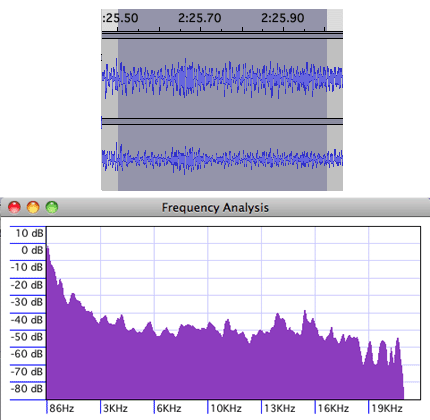# discrete Fourier transform

(redirected from Offset DFT)

## discrete Fourier transform

[di¦skrēt für·yā ′tranz‚fȯrm]
(mathematics)
A generalization of the Fourier transform to finite sets of data; for a function ƒ defined at N data values, 0, 1, 2, …, N - 1, the discrete Fourier transform is a function, ƒ, also defined on the set (0, 1, 2, …, N - 1, the discrete Fourier transform is a function, ƒ, also defined on the set (0, 1, 2, …, N - 1), whose value at n is the sum over the variable r, from 0 through N-1, of the quantity N -1ƒ(r) exp (-inr / N).
McGraw-Hill Dictionary of Scientific & Technical Terms, 6E, Copyright © 2003 by The McGraw-Hill Companies, Inc.

## discrete Fourier transform

(mathematics)
(DFT) A Fourier transform, specialized to the case where the abscissas are integers.

The DFT is central to many kinds of signal processing, including the analysis and compression of video and sound information.

A common implementation of the DFT is the Fast Fourier Transform (FFT).

## FFT

(Fast Fourier Transform) A computer algorithm used in digital signal processing (DSP) to modify, filter and decode digital audio, video and images. FFTs commonly change the time domain into the frequency domain.

An FFT TransformA Fourier transform was used to chart the power levels at different frequencies from the half second of digital samples (top).